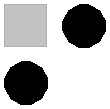## Quiz Number 5

This is the fifth quiz given in CPSC 124: Introductory Programming, Winter 1998. See the information page for that course for more information.

Question 1: The definition of an applet named MyApplet would begin with the line

class MyApplet extends Applet {

What is going on here? What is "Applet"? What is the word "extends" doing there?

Answer: Applet is the name of a class that is a standard part of Java. Here, MyApplet is a new class that is being defined as a subclass of Applet. The word "extends" is used to create subclasses in this way. The class MyApplet inherits everything that is in the class Applet. The rest of the definition of MyApplet will consist of additions and modifications to the stuff that is inherited from Applet.

(Note: The Applet class is actually defined in the package java.applet, so the above line has to be preceded by "import java.applet.*" or "import java.applet.Applet;" to make the applet class available.)

Question 2: Assume that g is an object belonging to the Graphics class. What image would be produced by the following commands? Draw a picture as part of your answer.

```           g.setColor(Color.gray);
g.fillRect(0,0,40,40);
g.fillRect(50,50,0,0);
g.setColor(Color.black);
g.fillOval(50,0,40,40);
g.fillOval(0,50,40,40);
```

Answer: The method "g.fillRect(x,y,w,h)" draws a solid rectangle with its upper left corner at the point (x,y). The other two parameters, w and h, give the width and height of the rectangle. In the second line "g.fillRect(50,50,0,0)" doesn't produce any image at all, since the width and height are specified as zero. The method "g.fillOval(x,y,w,h)" draws a solid oval that just fits inside the rectangle with upper left corner at (x,y) and with width w and height h. So, the picture produced contains one gray square and two black circles, arranged like this:(Note: I actually meant the second line to be "g.fillRect(50,50,40,40)." Without this typo, the picture would have another gray square.)

Question 3: An applet can define a method

public boolean keyDown(Event evt, int key)

What is the purpose of such a method? What is the key parameter for? Why is this method "public"?

Answer: This keyDown method will be called by the "system" when the user presses a key on the keyboard (assuming that the applet has the input focus at that time). You would write such a method to react to the user's keystrokes. The key parameter tells which key the user pressed. This method has to be public since it is called by the system, from outside the class that contains the method.

Question 4: A variable can be declared to be final, as in the declaration "static final int ballCount = 25;". What does it mean to declare the variable to be "final"? Why would you want to use such a variable in a program? (For extra credit: Why do you suppose that "final" variables are generally declared to be "static" as well?)

Answer: When a variable is declared to be "final," its value can never be changed from the value that is initially assigned to it. The variable is said to be a named constant. The two main reasons for using final variables are:

• Using a meaningful name such as "ballCount" to represent a quantity, rather than an actual value such as 25, makes the program easier to read and understand.
• Supose you want to modify your program to use a different value for the constant (for example, ballCount = 50 instead of ballCount = 25). It is easy to change the value of a final variable in the single line where it is declared. In general, it would be much harder to find and change an actual value every place it occurs in the program.

Extra credit: If a variable is non-static, then every object that belongs to the class gets its own copy of the variable. In the case of a final variable, all these copies would have the same value. It makes more sense to have a single copy, associated with the class. So final variables are usually declared to be static.

Question 5: Explain what is meant by polymorphism, and give an example.

Answer: Polymorphism refers to the fact that a method call, such as shape.draw(), can call different methods, depending on the actual type of the object. If the variable, shape, is declared to be of type Shape, then it can refer to an object belonging to the class Shape or to any subclass of that class. Each of these subclasses might have its own version of the draw() method. The method that is actually called by the command shape.draw() depends on which class the shape object actually belongs to.

David Eck, 20 February 1998